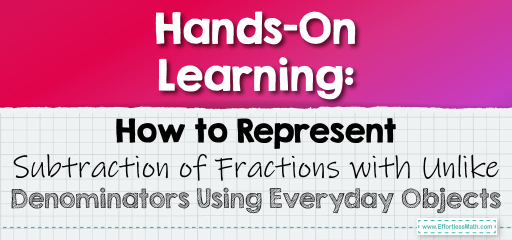# Hands-On Learning: How to Represent Subtraction of Fractions with Unlike Denominators Using Everyday Objects

Subtracting fractions with different denominators can sometimes be a tricky concept to grasp. However, by using tangible objects from our daily lives, we can visualize and better understand the process.This blog post will guide you through representing the subtraction of fractions with unlike denominators using common objects.

## Step-by-step Guide:

1. Grasping the Basics:

To subtract fractions, it’s essential to have a common denominator. This ensures that we’re taking away parts of the same size.

2. Selecting Suitable Objects:

Pick objects that can be easily segmented, such as pieces of fruit, toy blocks, or sections of a chocolate bar. Ensure you have enough to represent the denominators of the fractions you’re addressing.

3. Segmenting the Objects:

Divide your objects based on the denominators of the fractions. For instance, if working with $$\frac{3}{5}$$ and $$\frac{1}{10}$$, split an apple into 5 equal parts and a chocolate bar into 10 equal sections.

4. Identifying a Common Denominator with Objects:

The fractions need the same denominator for subtraction. Using the objects, determine a size that both sets can be adjusted to. In our example, 10 is the common denominator since both 5 and 10 can be adjusted to it.

5. Representing the Fractions:

Use the objects to depict each fraction. For $$\frac{3}{5}$$, take three parts out of the five. For $$\frac{1}{10}$$, take one part out of the ten.

6. Subtracting Using Objects:

Remove the parts of the second fraction from the first. Count the remaining parts and represent them over the common denominator. In our example, you’ll have 2 parts left out of 10, symbolizing $$\frac{2}{10}$$, which can be simplified to $$\frac{1}{5}$$.

### Example 1:

Subtract $$\frac{1}{6}$$ from $$\frac{4}{12}$$ using toy blocks.

Solution:

With a set of blocks divided into 6 and another into 12, the common denominator is 12. Representing the fractions, you’ll have 2 blocks for $$\frac{1}{6}$$ and 4 blocks for $$\frac{4}{12}$$. After subtraction, you’re left with 2 blocks out of 12, or $$\frac{2}{12}$$, which simplifies to $$\frac{1}{6}$$.

The Absolute Best Book for 5th Grade Students

### Example 2:

Subtract $$\frac{2}{8}$$ from $$\frac{3}{4}$$ using pieces of fruit.

Solution:

If you divide a fruit into 8 parts and another into 4 parts, the common denominator is 8. Representing the fractions, you’ll have 6 parts for $$\frac{3}{4}$$ and 2 parts for $$\frac{2}{8}$$. After subtraction, you have 4 parts out of 8, or $$\frac{4}{8}$$, which simplifies to $$\frac{1}{2}$$.

### Practice Questions:

1. Subtract $$\frac{2}{7}$$ from $$\frac{3}{14}$$ using chocolate bars.

2. Subtract $$\frac{1}{9}$$ from $$\frac{2}{3}$$ using toy blocks.

3. Subtract $$\frac{3}{10}$$ from $$\frac{1}{5}$$ using pieces of fruit.

A Perfect Book for Grade 5 Math Word Problems!

1. $$\frac{-1}{14}$$

2. $$\frac{4}{9}$$

3. $$\frac{-1}{10}$$

The Best Math Books for Elementary Students

### What people say about "Hands-On Learning: How to Represent Subtraction of Fractions with Unlike Denominators Using Everyday Objects - Effortless Math: We Help Students Learn to LOVE Mathematics"?

No one replied yet.

X
51% OFF

Limited time only!

Save Over 51%

SAVE $15 It was$29.99 now it is \$14.99